# The Hungarian RingsThe Hungarian Rings or Devil's Circles is a sliding puzzle consisting of two interwoven rings, each of which has several (usually 2x9 + 2x10) color-labeled pieces you can't take out. Moving the balls around the circles can switch balls, rearranging their order. The challenge is to set the balls so the corresponding colors are next to each other. There are some variations where more than two circles are used (Olympics Logo with 5 circles) or the two circles are not plane or the balls are marked by numbers not by colors.
The Hungarian rings puzzle seems to be easy but it is not really so straight forward. After you solve the firs two colors (picture) you can get stuck and may need some help. Here I show you the easiest solution of the Hungarian Rings Puzzle where you have to learn only a single algorithm.

## Hungarian Rings solution

 Every state is considered to be a valid solution where the 4 colors are coherent. On the picture is one example of the solved state. In my case I have 10 pieces of red, 10 black, 9 yellow and 9 blue balls. Different models can have other color scheme but in this tutorial we're going to demonstrate the solution with these colors.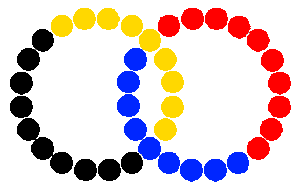First of all solve intuitively one set of colors which has 10 balls (black) in the left ring, then the other set of 10 balls (red) in the right. This part of the Hungarian Ring solution is intuitive and quite easy, don't need explication. When this is done try to arrange the inner colors so in the two intersections of the rings have different colors (yellow and blue balls).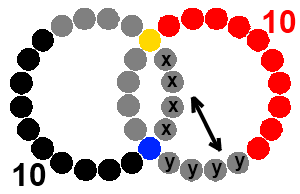In the solution shown below you can switch one of the X-marked balls with one of the Y-marked balls. To switch two pieces in the upper part just turn the puzzle upside down and follow the same steps. Let's say we want to switch the A and B balls not messing up the rest of the puzzle.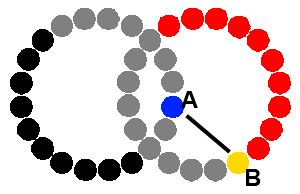1. Turn the left ring counterclockwise until the A ball is the fifth after the upper intersection.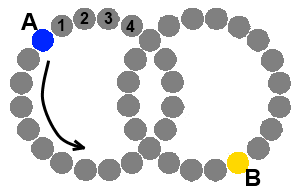2. Turn the right circle until the B piece reaches the upper intersection.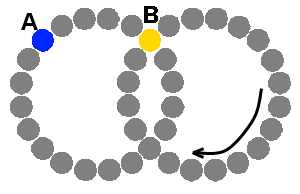3. Move the left circle until the A ball gets to the upper and the B gets to the bottom intersection.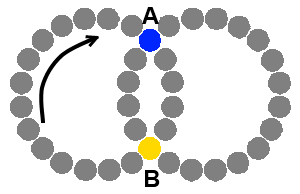4. Turn the right ring clockwise 5 steps until the B piece reaches the upper intersection.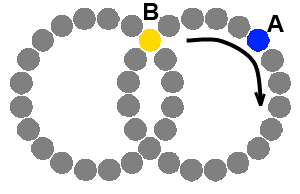5. Now rotate the left ring until the red balls get next to each other.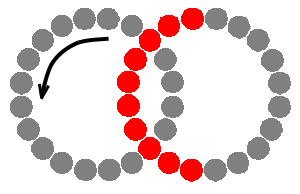6. Just like in the previous step rotate the right ring until the black balls come together.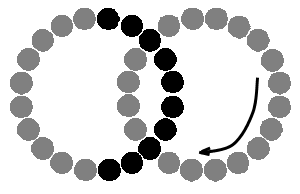7. Turn the left side to align the black balls next to the bottom intersection.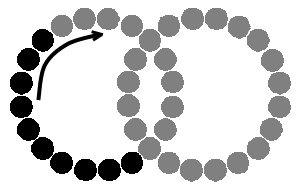Repeat switching the blue and yellow balls until your Hungarian Rings puzzle is fully solved.